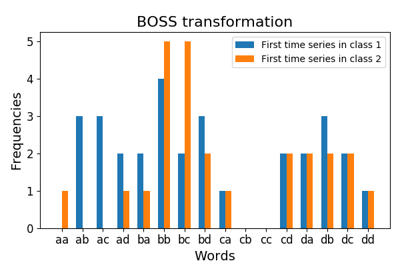# `pyts.transformation`.BOSS¶

class `pyts.transformation.``BOSS`(word_size=4, n_bins=4, strategy='quantile', window_size=10, window_step=1, anova=False, drop_sum=False, norm_mean=False, norm_std=False, numerosity_reduction=True, sparse=True, alphabet=None)[source]

Bag of Symbolic Fourier Approximation Symbols.

For each time series, subseries are extracted using a slidind window. Then the subseries are transformed into a word using the Symbolic Fourier Approximation (SFA) algorithm. For each time series, the words are grouped together and a histogram counting the occurences of each word is created.

Parameters: word_size : int (default = 4) Size of each word. n_bins : int (default = 4) The number of bins to produce. It must be between 2 and 26. strategy : str (default = ‘quantile’) Strategy used to define the widths of the bins: ‘uniform’: All bins in each sample have identical widths ‘quantile’: All bins in each sample have the same number of points ‘normal’: Bin edges are quantiles from a standard normal distribution ‘entropy’: Bin edges are computed using information gain window_size : int or float (default = 10) Size of the sliding window. If float, it represents the percentage of the size of each time series and must be between 0 and 1. The window size will be computed as `ceil(window_size * n_timestamps)`. window_step : int or float (default = 1) Step of the sliding window. If float, it represents the percentage of the size of each time series and must be between 0 and 1. The window size will be computed as `ceil(window_step * n_timestamps)`. anova : bool (default = False) If True, the Fourier coefficient selection is done via a one-way ANOVA test. If False, the first Fourier coefficients are selected. drop_sum : bool (default = False) If True, the first Fourier coefficient (i.e. the sum of the subseries) is dropped. Otherwise, it is kept. norm_mean : bool (default = False) If True, center each subseries before scaling. norm_std : bool (default = False) If True, scale each subseries to unit variance. numerosity_reduction : bool (default = True) If True, delete sample-wise all but one occurence of back to back identical occurences of the same words. sparse : bool (default = True) Return a sparse matrix if True, else return an array. alphabet : None, ‘ordinal’ or array-like, shape = (n_bins,) Alphabet to use. If None, the first n_bins letters of the Latin alphabet are used.

References

 [R1d415568a83d-1] P. Schäfer, “The BOSS is concerned with time series classification in the presence of noise”. Data Mining and Knowledge Discovery, 29(6), 1505-1530 (2015).

Examples

```>>> from pyts.datasets import load_gunpoint
>>> from pyts.transformation import BOSS
>>> X_train, X_test, _, _ = load_gunpoint(return_X_y=True)
>>> boss = BOSS(word_size=2, n_bins=2, sparse=False)
>>> boss.fit(X_train) # doctest: +ELLIPSIS
BOSS(...)
>>> sorted(boss.vocabulary_.values())
['aa', 'ab', 'ba', 'bb']
>>> boss.transform(X_test) # doctest: +ELLIPSIS
array(...)
```
Attributes: vocabulary_ : dict A mapping of feature indices to terms.

Methods

 `__init__`(self[, word_size, n_bins, …]) Initialize self. `fit`(self, X[, y]) Fit the model according to the given training data. `fit_transform`(self, X[, y]) Fit the data then transform it. `get_params`(self[, deep]) Get parameters for this estimator. `set_params`(self, \*\*params) Set the parameters of this estimator. `transform`(self, X) Transform the provided data.
`__init__`(self, word_size=4, n_bins=4, strategy='quantile', window_size=10, window_step=1, anova=False, drop_sum=False, norm_mean=False, norm_std=False, numerosity_reduction=True, sparse=True, alphabet=None)[source]

Initialize self. See help(type(self)) for accurate signature.

`fit`(self, X, y=None)[source]

Fit the model according to the given training data.

Parameters: X : array-like, shape = (n_samples, n_timestamps) Training vector. y : None or array-like, shape = (n_samples,) Class labels for each data sample. self : object
`fit_transform`(self, X, y=None)[source]

Fit the data then transform it.

Parameters: X : array-like, shape = (n_samples, n_timestamps) Training vector. y : None or array-like, shape = (n_samples,) Class labels for each data sample. X_new : sparse matrix, shape = (n_samples, n_words) Document-term matrix.
`get_params`(self, deep=True)

Get parameters for this estimator.

Parameters: deep : bool, default=True If True, will return the parameters for this estimator and contained subobjects that are estimators. params : mapping of string to any Parameter names mapped to their values.
`set_params`(self, **params)

Set the parameters of this estimator.

The method works on simple estimators as well as on nested objects (such as pipelines). The latter have parameters of the form `<component>__<parameter>` so that it’s possible to update each component of a nested object.

Parameters: **params : dict Estimator parameters. self : object Estimator instance.
`transform`(self, X)[source]

Transform the provided data.

Parameters: X : array-like, shape = (n_samples, n_timestamps) Test samples. X_new : sparse matrix, shape = (n_samples, n_words) Document-term matrix.

## Examples using `pyts.transformation.BOSS`¶Bag-of-SFA Symbols (BOSS)